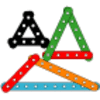You may also likeI'm Eight

Find a great variety of ways of asking questions which make 8.Let's Investigate Triangles

Vincent and Tara are making triangles with the class construction set. They have a pile of strips of different lengths. How many different triangles can they make?Noah

Noah saw 12 legs walk by into the Ark. How many creatures did he see?

Secret Number

Age 5 to 7 Challenge Level:

Annie added $4$ to her number and it made $10$. What number added to $4$ makes $10$?
When Charlie "timesed" his number by $5$, it came to $35$. What number multiplied by $5$ makes $35$?